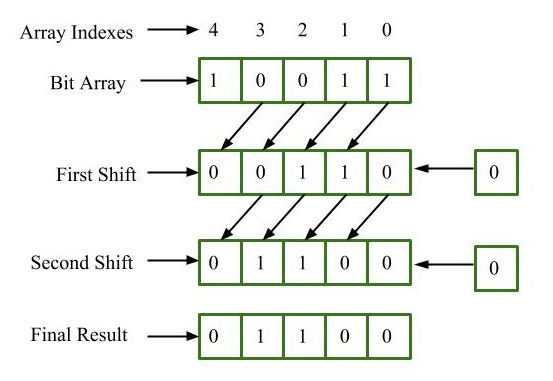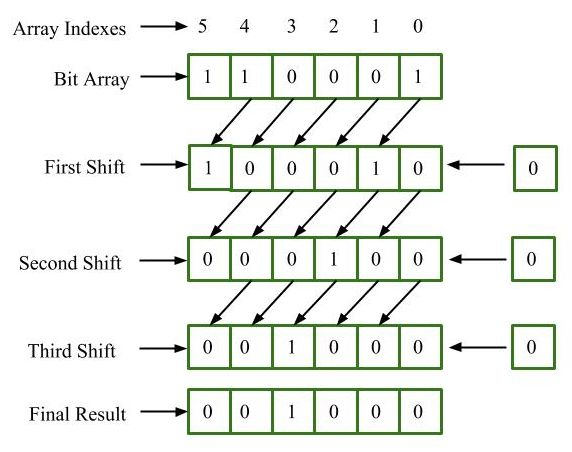Related Articles

# BitArray.LeftShift() Method in C# with Examples

• Last Updated : 27 Mar, 2019

BitArray class manages a array of bit values, which are represented as Booleans, where true indicates bit is 1 and false indicates bit is 0. This class is contained in namespace, System.Collections. BitArray.LeftShift(Int32) method is used to shift the bits of the bit array to the left by one position and adds zeros on the shifted position. Original BitArray object will be modified on performing the operation Left shift.

Syntax: public System.Collections.BitArray LeftShift (int count);

Parameter:
count is an immutable value type that represents signed integers with values that range from negative 2,147,483,648 through positive 2,147,483,647.

Return value : It returns Bit Array.

Example 1: Suppose we have the bit array 10011 we want to shift it Left by two positions.The final result is 00110.

 `// C# program to illustrate the``// BitArray.LeftShift() Method``using` `System;``using` `System.Collections;`` ` `class` `GeeksforGeeks {`` ` `    ``// Main Method``    ``public` `static` `void` `Main()``    ``{`` ` `        ``// Creating a BitArray``        ``BitArray BitArr = ``new` `BitArray(5);`` ` `        ``// Initializing values in BitArr``        ``BitArr = ``true``;``        ``BitArr = ``true``;``        ``BitArr = ``false``;``        ``BitArr = ``false``;``        ``BitArr = ``true``;`` ` `        ``// function calling``        ``Display(BitArr.LeftShift(2));``    ``}`` ` `    ``// Displaying the result``    ``public` `static` `void` `Display(IEnumerable myList)``    ``{``        ``foreach``(Object obj ``in` `myList)``        ``{``            ``Console.WriteLine(obj);``        ``}``    ``}``}`
Output:
```False
False
True
True
False
```

Example 2: Suppose we have the bit array 100011 we want to shift it left by three positions.The final result is 000100.

 `// C# program to illustrate the``// BitArray.LeftShift() Method``using` `System;``using` `System.Collections;`` ` `class` `GeeksforGeeks {`` ` `    ``// Main Method``    ``public` `static` `void` `Main()``    ``{`` ` `        ``// Creating a BitArray``        ``BitArray BitArr = ``new` `BitArray(6);`` ` `        ``// Initializing values in BitArr``        ``BitArr = ``true``;``        ``BitArr = ``false``;``        ``BitArr = ``false``;``        ``BitArr = ``false``;``        ``BitArr = ``true``;``        ``BitArr = ``true``;`` ` `        ``// function calling``        ``Display(BitArr.LeftShift(3));``    ``}`` ` `    ``// Displaying the result``    ``public` `static` `void` `Display(IEnumerable myList)``    ``{``        ``foreach``(Object obj ``in` `myList)``        ``{``            ``Console.WriteLine(obj);``        ``}``    ``}``}`
Output:
```False
False
False
True
False
False
```

Reference:

My Personal Notes arrow_drop_up